SohCahToa: Definition & Example Problems

Lesson Transcript
Instructor
Stephanie Matalone

Stephanie taught high school science and math and has a Master's Degree in Secondary Education.

Expert Contributor
Robert Ferdinand

Robert Ferdinand has taught university-level mathematics, statistics and computer science from freshmen to senior level. Robert has a PhD in Applied Mathematics.

In mathematics, SOHCAHTOA is a mnemonic device to help students learn trigonometry's three primary functions: sine, cosine, and tangent. Explore the definition of SOHCAHTOA, and review example problems using SOHCAHTOA to help calculate ratios and lengths. Updated: 08/31/2021

Trigonometric Functions

Sine, cosine, and tangent are the three main functions in trigonometry. They're all based on ratios obtained from a right triangle. Before we can discuss what ratios work for which function, we need to label a right triangle.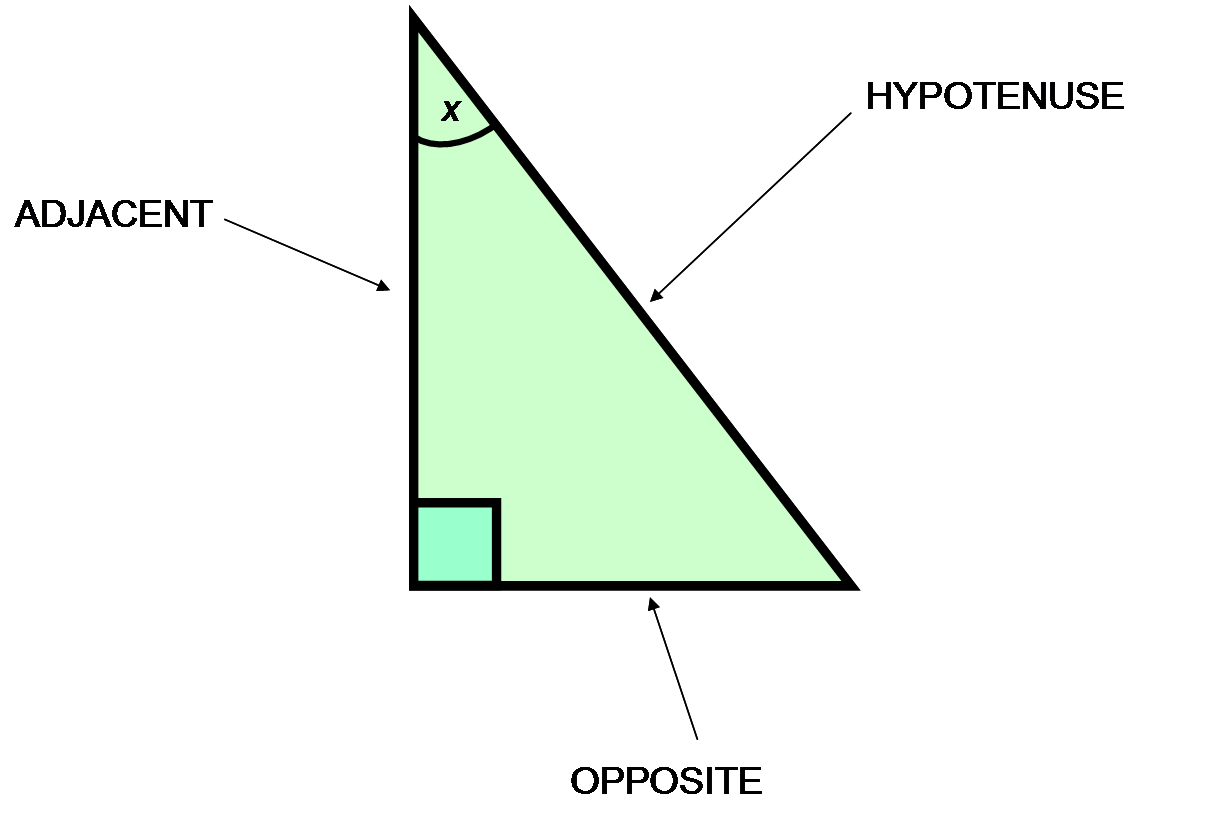Opposite is the side opposite the angle in question, adjacent is the side next to the angle in question, and the hypotenuse is the longest side of a right triangle. The hypotenuse is always opposite the right angle.

The ratios that allow you to determine the sine, cosine, and tangent of a right triangle are:

• The sine of an angle is equal to the side opposite the angle divided by the hypotenuse.
• The cosine of an angle is equal to the side adjacent to the angle divided by the hypotenuse.
• The tangent of an angle is equal to the side opposite the angle divided by the side adjacent to the angle.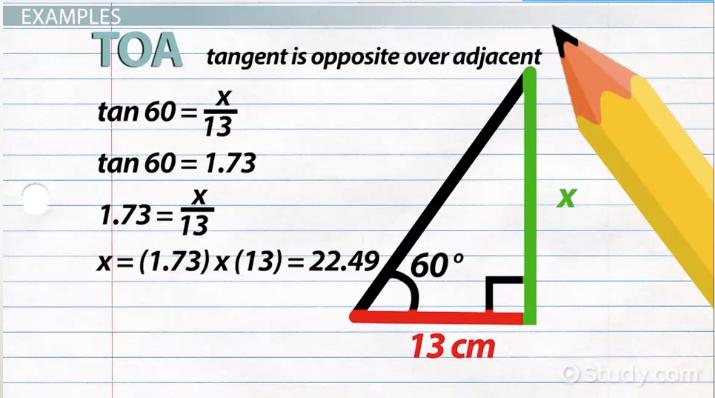An error occurred trying to load this video.

Try refreshing the page, or contact customer support.

Coming up next: Special & Common Trig Values: Explanation & Overview

You're on a roll. Keep up the good work!

Replay
Your next lesson will play in 10 seconds
• 0:02 Trigonometric Functions
• 0:51 SohCahToa
• 1:31 Examples
• 2:55 Real-Life Examples
• 4:14 Lesson Summary
Save Save

Want to watch this again later?

Timeline
Autoplay
Autoplay
Speed Speed

SOHCAHTOA

These ratios can be difficult to remember. You might easily get confused and not remember which side goes where. SOHCAHTOA is a mnemonic device helpful for remembering what ratio goes with which function.

• SOH = Sine is Opposite over Hypotenuse
• CAH = Cosine is Adjacent over Hypotenuse
• TOA = Tangent is Opposite over Adjacent

With these properties, you can solve almost any problem related to finding either a side length or angle measure of a right triangle. SohCahToa can ensure that you won't get them wrong.

Examples

Now, for some example problems. Let's find x for this triangle.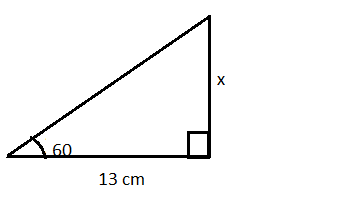We know the side adjacent to the known angle of 60 degrees is 13 cm. We're trying to find the length of the side opposite the known angle of 60 degrees. Thus, we need to use TOA, or tangent (tan), which uses opposite and adjacent. Therefore, our equation will be:

tan 60 = x/13

The tan of 60 is 1.73, which makes the equation:

1.73 = x/13

Solve for x to get:

x = (1.73) * (13) = 22.49

So, the length of side x is 22.49 cm.

On to another example problem. What is the sine of 35 degrees?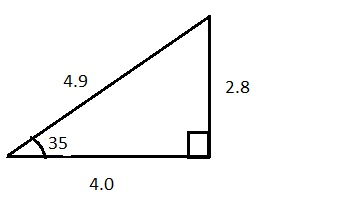The sine of an angle is equal to the opposite side divided by the hypotenuse.

sin 35 = 2.8 / 4.9 = 0.57

And for one final problem, find sin, cos and tan for the angle in the right triangle shown.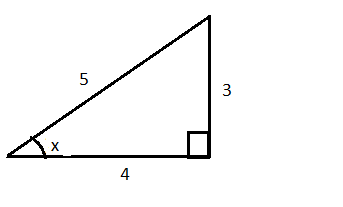sin = opposite/hypotenuse = 3 / 5 = 0.6

cos = adjacent/hypotenuse = 4 / 5 = 0.8

tan = opposite/adjacent = 3 / 4 = 0.75

Real-Life Examples

Trigonometric functions are important for many reasons. They let you calculate angles when you know sides and calculate sides when you know angles. This can be helpful in many real life situations, such as when determining the height of a large building or the distance across a lake - things that just can't be measured easily.

To unlock this lesson you must be a Study.com Member.

Practice Questions

1. For the triangle ABC shown below, use SohCahToa to find side AB, side BC. Then write down the measure of angle B.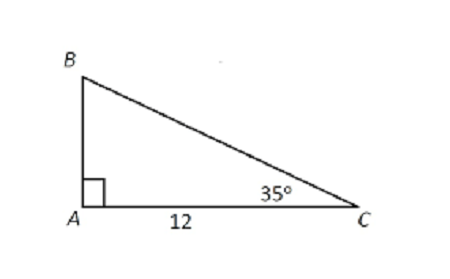2. A rope is attached to the top of a vertical, straight tree. The other end of the rope is tied to a point on the level ground at a distance of 20 feet from the base of the tree which is also on level ground. The rope makes an angle of 60 degrees with the level ground. Use SohCahToa to calculate the height of the tree.

1. Using Toa we define

tan 35 = Opposite/Adjacent = AB/AC = AB/12.

Then cross multiplying the above relation gives us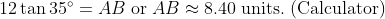Next, using Cah we get

cos 35 = Adjacent/Hypotenuse = AC/BC = 12/BC.

Cross multiplying the above relation leads to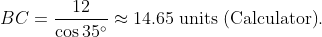Finally, since ABC is a right triangle with angle A = 90 degrees and angle C = 35 degrees, angle B will equal 180 - (90 + 35) = 180 - 125 = 55 degrees.

Hence, AB = 8.40 units, BC = 14.65 units and angle B = 55 degrees.

2. Let the top of the rope attached to the top of the tree be point A. Then let B be the base of the tree. Finally let C be the point where the rope is attached to the level ground.

Since AB is perpendicular to BC, therefore ABC is a right triangle.

Next, BC = 20 feet and angle C = 60 degrees.

Hence to find the height of the tree or AB, we use Toa in the triangle ABC to get

tan C = Opposite/Adjacent = AB/BC

OR

tan 60 = AB/20.

Cross multiplying the above relation yields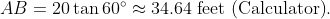The height of the tree is 34.64 feet.

Register to view this lesson

Are you a student or a teacher?